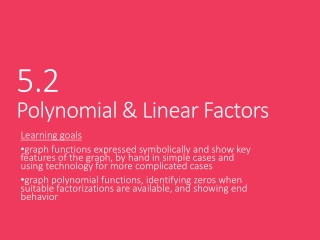DownloadDownload Presentation5.2 Polynomial & Linear Factors

5.2 Polynomial & Linear Factors

Télécharger la présentation5.2 Polynomial & Linear Factors

- - - - - - - - - - - - - - - - - - - - - - - - - - - E N D - - - - - - - - - - - - - - - - - - - - - - - - - - -
Presentation Transcript

1. 5.2 Polynomial & Linear Factors Learning goals graph functions expressed symbolically and show key features of the graph, by hand in simple cases and using technology for more complicated cases graph polynomial functions, identifying zeros when suitable factorizations are available, and showing end behavior

2. Ex 1 Write in factored form, and then find the zeros.

3. The following are equivalent statements about a real number b and a polynomial P(x): • (x – b) is a factor of P(x) • b is a zero of the function • b is a root (or solution) of P(x) = 0 • b is an x-intercept of the graph

4. So, for example… Equivalent Statements about Polynomials • – 4 is a solution of • – 4 is an x-intercept of the graph • – 4 is a zeroof • (x + 4) is a factor of

5. Ex 2 Find the zeros and graph

6. Ex 3 Find the zeros and graph

7. Ex 4 Write an equation in standard form with zeros at

8. Vocabulary multiple zero: a repeated zero of a polynomial that occurs because of a repeated linear factor multiplicity : the number of times a zero occurs in a polynomial

9. Ex 5 For the following polynomial, find any multiple zeros and state the multiplicity of each

10. Vocabulary • relative minimumand relative maximum : peaks and valleys within the graph of a polynomial that has several turning points

11. Ex 5 Find the relative minimum and maximum